Ch 5. Rigid Body General Motion Multimedia Engineering Dynamics Fixed Axis Rotation Plane Motion Velocities Zero Velocity Point Plane Motion Accelerations Multiple Gears Rot. Coord. Velocities Rot. Coord. Acceleration
 Chapter - Particle - 1. General Motion 2. Force & Accel. 3. Energy 4. Momentum - Rigid Body - 5. General Motion 6. Force & Accel. 7. Energy 8. Momentum 9. 3-D Motion 10. Vibrations Appendix Basic Math Units Basic Equations Sections Search eBooks Dynamics Fluids Math Mechanics Statics Thermodynamics Author(s): Kurt Gramoll ©Kurt GramollSTATICS - CASE STUDY SOLUTION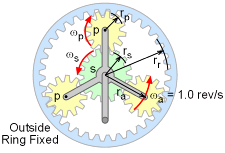Gear Assembly s = sun, p = planet a = arm, r = ring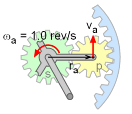Center Velocity of Planet Gear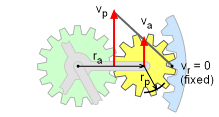Inside Velocity of Planet Gear The objective in designing the salad spinner is to maximize the spin rate of the inside bowl (connect to the sun gear) for a given handle (or arm) angular velocity. The epicyclical gear mechanism has four different objects that can rotate. For the salad spinner, the outside ring gear is fixed and the arm or handle angular velocity is given as 1 rev/s. This leaves two other gears with unknown angular velocities, the sun and planet gears. Since the handle mechanism rotates about its center and its angular velocity is known, the linear velocity of the arm tip, va, is      va = ωa ra However, this is also the linear velocity of the planet gear center. Because the ring gear does not rotate, the velocity at the point of contact between the planet gear and the ring gear is zero (outside edge). By using similar triangles, the edge velocity of the planet gear where it contacts the sun gear, vp, can be calculated as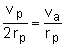vp = 2 va = 2(ωa ra)Velocity of Sun Gear \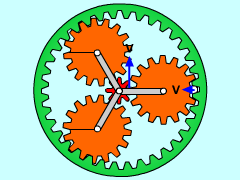Velocity at Point of Contact Between Gears Since the the planet gear and the sun gear are in contact (and do not slip), their tangent linear velocities must be equal, giving      vs = vp Since the sun gear is pinned, vs = rsωs. Substituting for vs and vp gives      ωsrs = 2 ωa ra      ωs = 2 ωa ra/rs The angular velocity magnification is dependent on the ratio of the carrier radius to the sun gear radius, ra/rs . A small sun gear and a large planet gear will produce the highest rotation rate for the bowl. Thus, the best radius for the sun gear ra is 0.5 inch (remember, there are only 4 gear sizes available). This requires the planet gear radius to be 1.5 inches.      ωa = 2 (1 rev/s) 1.5 in / 0.5 in = 6 rev/s This gives a speed magnification of 6 to 1.

Practice Homework and Test problems now available in the 'Eng Dynamics' mobile app
Includes over 400 problems with complete detailed solutions.
Available now at the Google Play Store and Apple App Store.